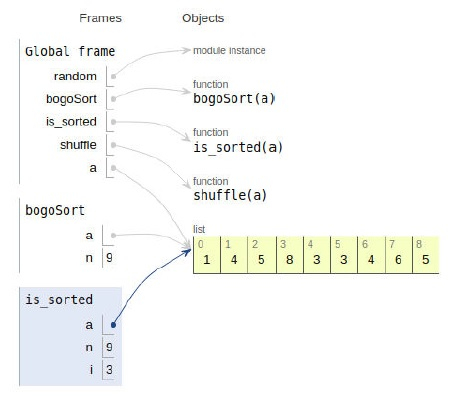# Python Program for BogoSort or Permutation Sort

Problem statement − We are given an array, we need to sort it using the concept of permutation sort.

BogoSort also is known as permutation sort, is based on generating and testing paradigms.

Now let’s observe the solution in the implementation below−

## Example

Live Demo

# random module
import random
# Sort
def bogoSort(a):
n = len(a)
while (is_sorted(a)== False):
shuffle(a)
# check
def is_sorted(a):
n = len(a)
for i in range(0, n-1):
if (a[i] > a[i+1] ):
return False
return True
# permutation
def shuffle(a):
n = len(a)
for i in range (0,n):
r = random.randint(0,n-1)
a[i], a[r] = a[r], a[i]
# main
a = [1,5,3,4,8,6,3,4,5]
bogoSort(a)
print("Sorted array :")
for i in range(len(a)):
print (a[i],end=" ")

## Output

Sorted array is :
1 3 3 4 4 5 5 6 8All the variables are declared in the local scope and their references are seen in the figure above.

## Conclusion

In this article, we have learned about how we can make a Python Program for BogoSort or Permutation Sort